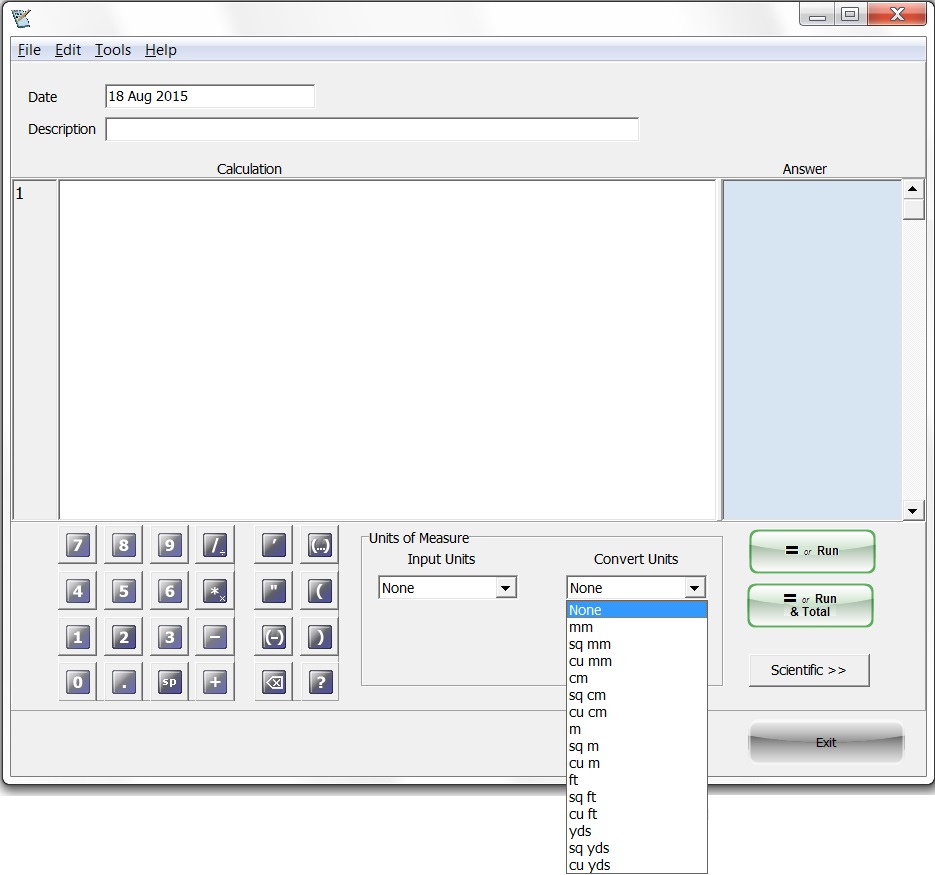# Exact Calculator Software

#### Exact Calculator - The Ultimate Visual and Programmable Calculation Pad

Exact Calculator is designed for those wanting to calculate in both Metric and English Units and keep a copy of the calculation for review and future reference

Exact Calculator is a great tool for Contractors and Estimators and any one who may wish to save and review their calculation at a later date

Exact Calculator allows you to see ALL your working calculations line by line

Features:

• Allows you to make corrections to the current calculation line or a previous line
• Subtotals and Totals
• Save your calculation and re-open it - add and/or correct it
• Convert millimetres/centimetres into metres, square metres and cubic metres
• Convert feet and inches into feet, square feet and cubic yards
• Contains advanced scientific functions sin, tan, cos...
• Fully programmable with a Visual Basic style interface

Example calculation of the volume of concrete in a floor slab surrounded by footings

• Calculation of Volume of East West footings x 2
• Calculation of Volume of North South footings x 2
• Calculation of Volume of floor slab
• Total Volume of concrete
• Calculation can be printed and saved for future reference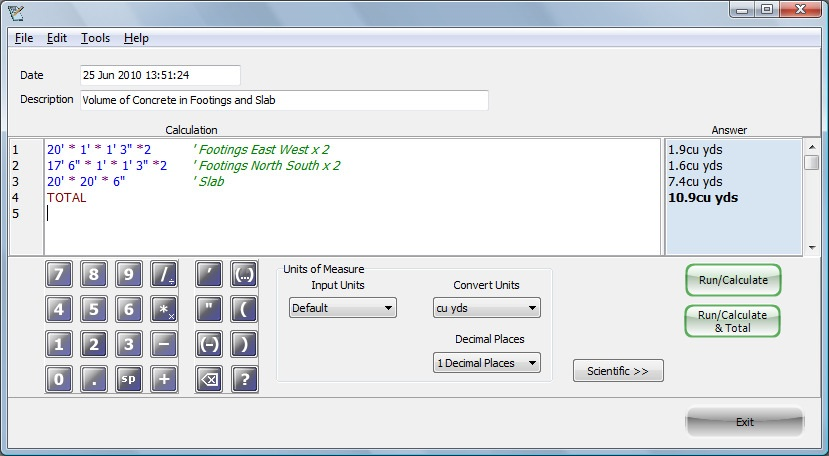Features:

• Simple Command Interface
• Keyboard entry
• Mouse Click entry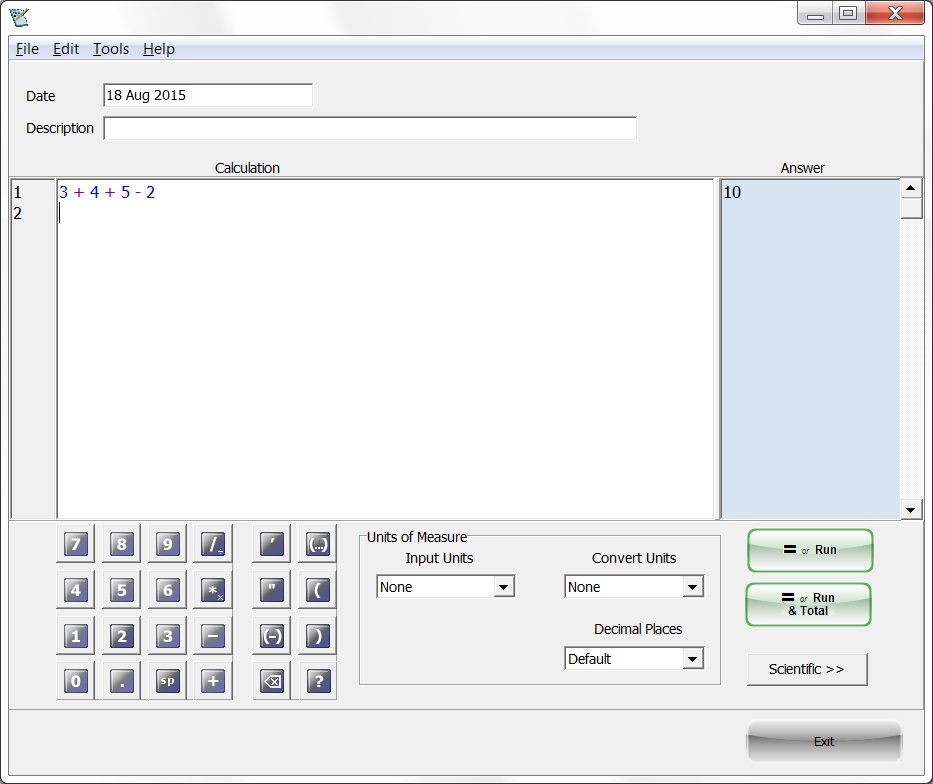Features:

• Simple multiplication and division
• In keeping with standard programming as there is no Multiply × or Divide ÷ symbol on the keyboard
• The asterisk * symbol is the multiply symbol
• The slash / symbol us the divide symbol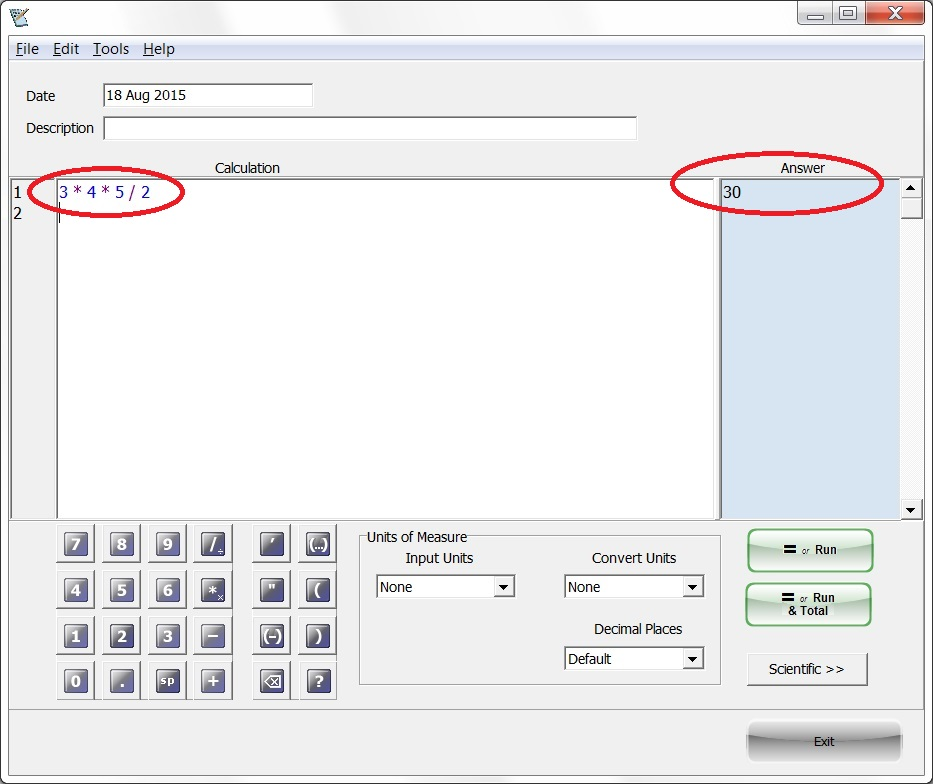Features:

• Multiple lines of calculation
• Grand Total for calculation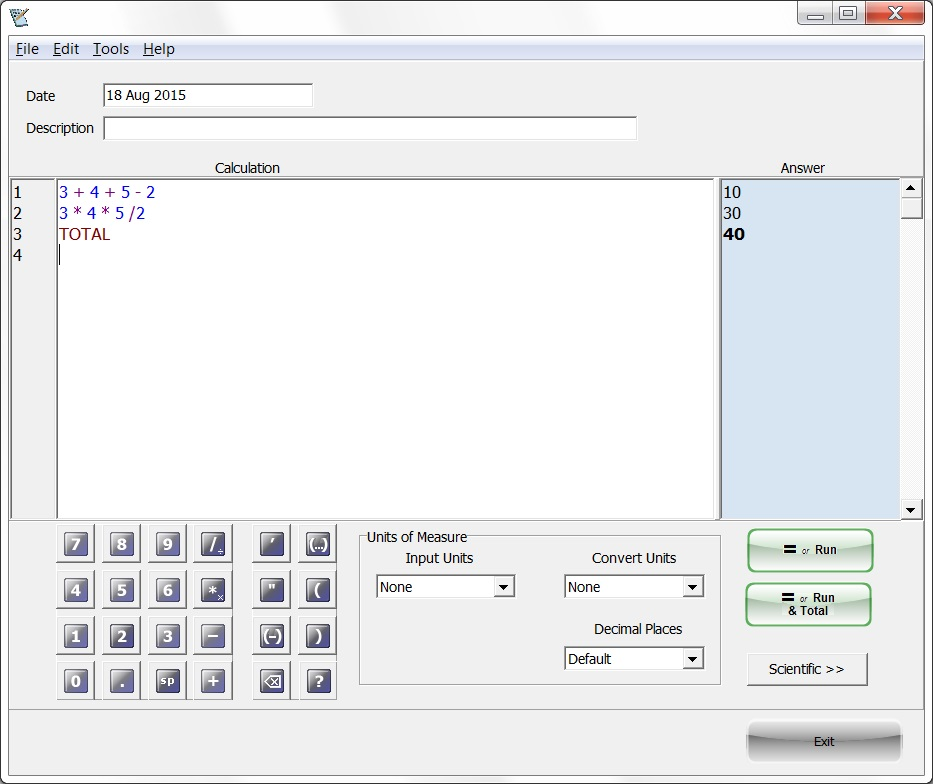Multiply 3 linear lengths and get answer in cubic feet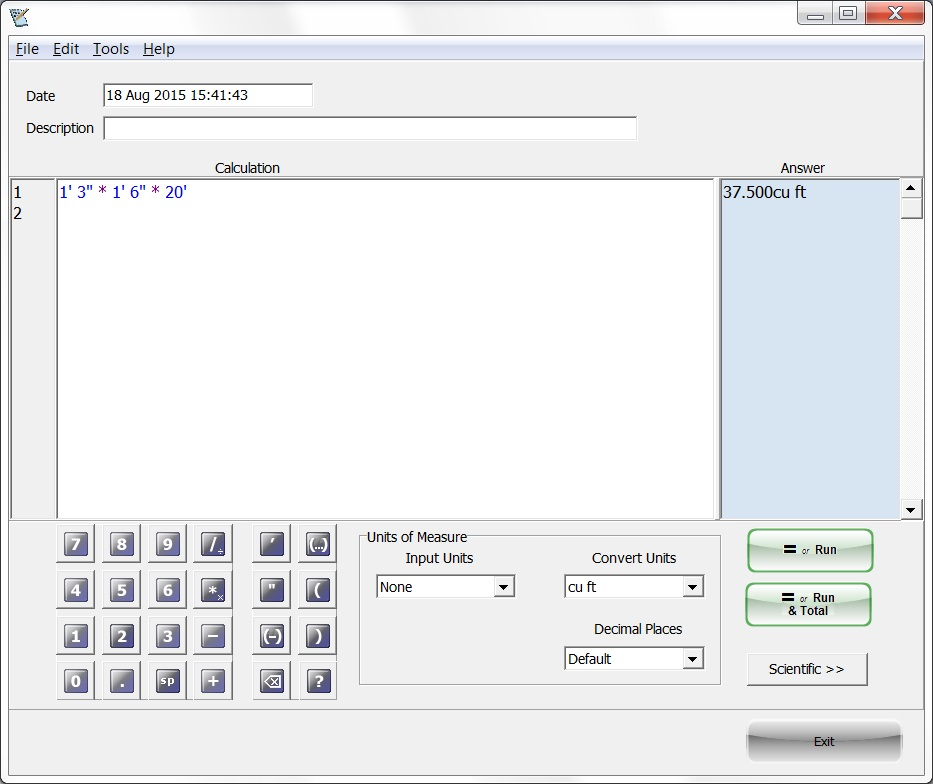Features:

• Multiplication of English Units
• Conversion of answer from cubic feet to cubic yards
• Total in Cubic Yards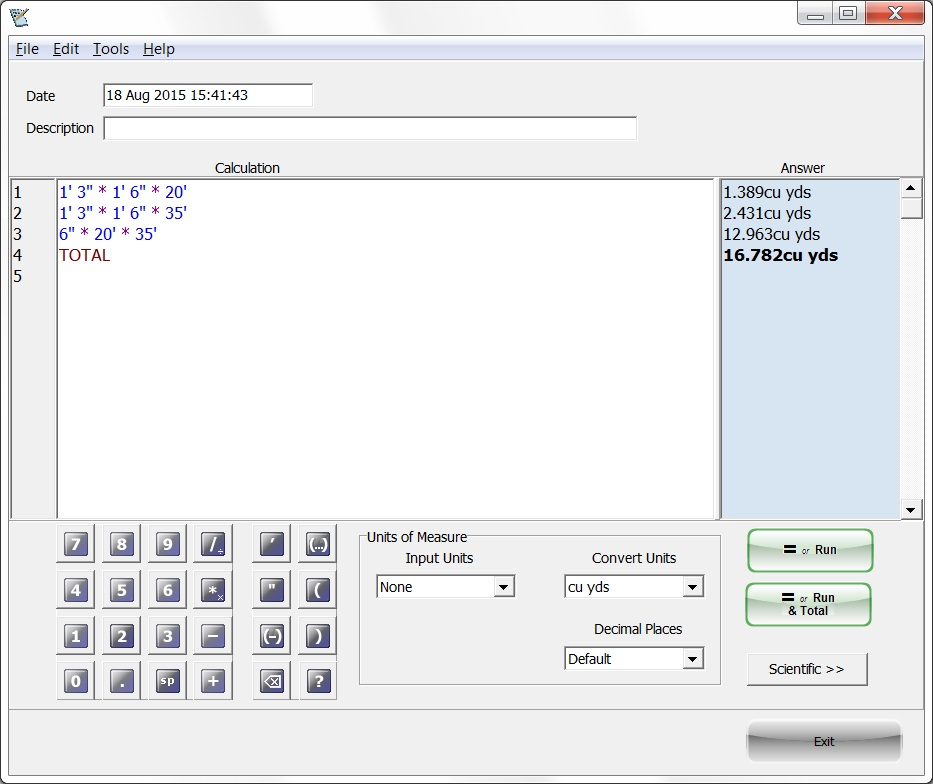Both English and Metric Units Available